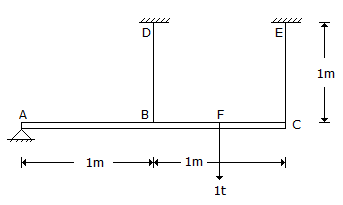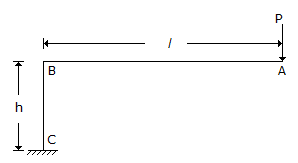# Civil Engineering - UPSC Civil Service Exam Questions

41.

ABC is rigid bar. It is hinged at A and suspended at B and C by two wires BD and CE made of copper and steel respectively, as shown in the given figure. The bar carries load of 1t at F, mid-way between B and C.

Given Ac = 4 cm2, A = 2 cm2, Ec = 1 x 106 kg/cm2, Es = 2 x 106 kg/cm2.

Ps is the force in the steel wire and Pc is the force in the copper wire, the ratio Pc/Ps will beA. 0.5 B. 4 C. 0.25 D. 2

Explanation:

No answer description available for this question. Let us discuss.

42.

A hydraulic model of a spillway is constructed with a scale 1 : 16. If the prototype discharge is 2048 cumec, then the corresponding discharge for which the model should be tested is

 A. 1 cumec B. 2 cumec C. 4 cumec D. 8 cumec

Explanation:

No answer description available for this question. Let us discuss.

43.

A beam has the same section throughout its length with I = 1 x 108mm4, It is subjected to a uniform B.M. = 40 kNm. E = 2 x 105 N/mm2, What is the radius of curvature of the circle into which the beam will bend in the form of an arc of a circle ?

 A. 1000 m B. 500 m C. 400 m D. 350 m

Explanation:

No answer description available for this question. Let us discuss.

44.

Consider the following statements in respect of plate girders :
1. A large number of cover plates are provided over flange angles so that curtailed flanges match the bending moment diagram exactly.
2. At least one cover plate should extend over the entire span so that rain water may not enter and corrode the connections.
3. A minimum of one-third of flange area should be provided in flange angles and balance in flange cover plates for stability.
Which of the statements given above are correct ?

 A. 1 and 2 B. 2 and 3 C. 1 and 3 D. 1, 2 and 3

Explanation:

No answer description available for this question. Let us discuss.

45.

A rigid cantilever frame ABC is fixed at C. It carries a load P at A, as shown in the given figure. Neglecting axial deformation, the vertical deflection of the point A is given by (EI constant throughout the frame).A. PIh2/2EI B. PI2h/3EI C. PI2(3h + I)/3EI D. PI2(2h + I)/2EI